### Confidence Intervals

A Confidence Interval is an interval of numbers containing the most plausible values for our Population Parameter. A point estimate gives a single value for a parameter. However, a point estimate is not perfect and usually there is some error in the estimate. Instead of giving just a point estimate of a parameter, it would be better to provide a range of values for the parameter. A plausible range of values for the population parameter is called a confidence interval.

So basically, we try to build the confidence interval around the point estimate. The probability that this procedure produces an interval that contains the actual true parameter value is known as the Confidence Level and is generally chosen to be 0.9, 0.95 or 0.99.

Confidence Intervals (for a population mean) take the form:

Point Estimate +/- Critical Value x Standard Error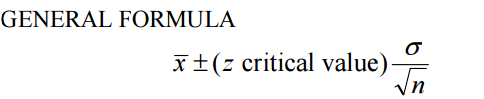Z-scores are appropriate confidence coefficients for a confidence interval of the mean when the population standard deviation (σ) is known.

The level of confidence determines the z critical value.

99%                                                                          2.58

95%                                                                            1.96

90%                                                                          1.645

###### Confidence Coefficients for 99% Confidence Interval from standard normal distribution: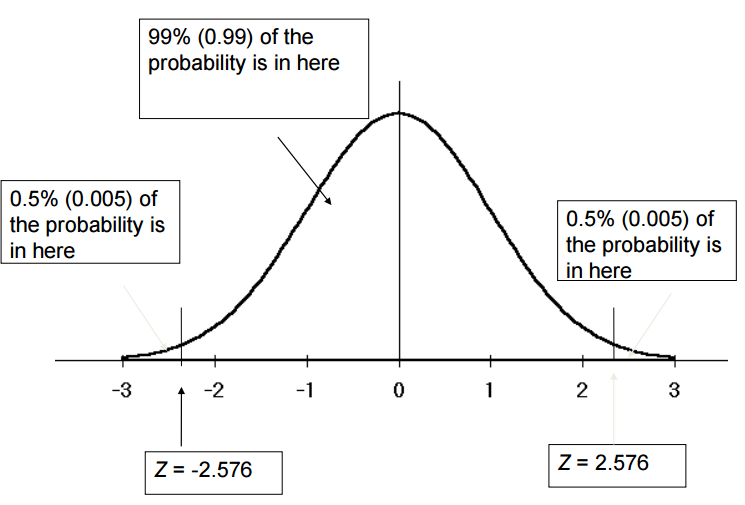###### Confidence Coefficients for 95% Confidence Interval from standard normal distribution: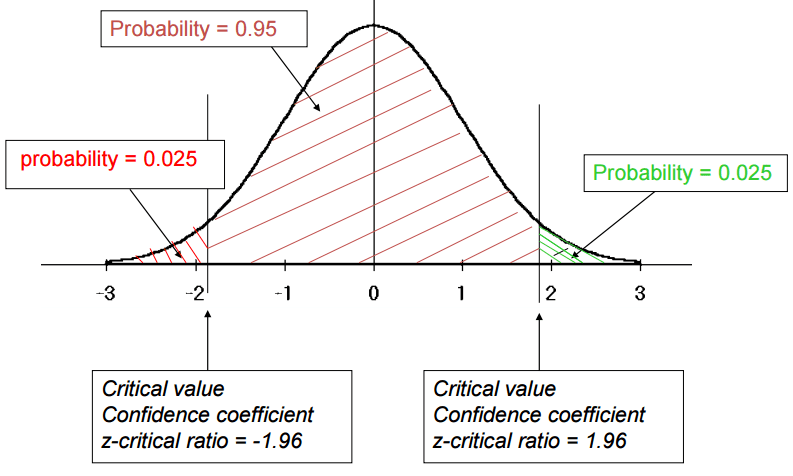###### Confidence Coefficients for 90% Confidence Interval from standard normal distribution:However, most of the time when the population mean is being estimated from sample data the population variance is unknown and must also be estimated from sample data. The sample standard deviation (s) provides an estimate of the population standard deviation (σ).

Since n is large the unknown σ can be replaced by the sample value s.The standard error represents the standard deviation associated with the estimate, and roughly 95% of the time the estimate will be within 2 standard errors of the parameter. If the interval spreads out 2 standard errors from the point estimate, we can be roughly 95% confident that we have captured the true parameter: point estimate ± 2 × SE. Similarly, we can construct 90% and 99% confidence interval using above z critical value.

###### Margin of error:

In a confidence interval, z × SE is called the margin of error.

#### Conditions for confidence interval for Population mean:

Some conditions need to be satisfied to use the above formula and to build the confidence interval. In fact, since this method is based on CLT it follows the same conditions for CLT.

• Independence: Sampled observations must be independent.
• Random sample/random assignment
• If sampling without replacement, then needs to be n < 10% of population.
• Sample size/ skew: Either the population distribution is normal, or if the population distribution is skewed, the sample size is large (rule of thumb: n > 30)

If sample size is less than 30 then we use t-distribution.

#### Example:

A random sample of 225 1st year statistics tutorials was selected from the past 5 years and the number of students absent from each one recorded. The results were=11.6 and s=4.1 absences. Estimate the mean number of absences per tutorial over the past 5 years with 90% confidence.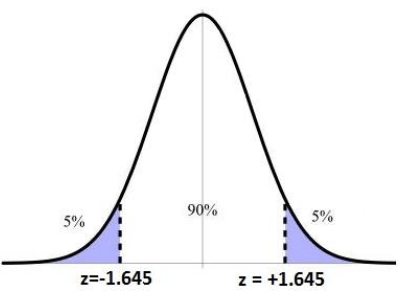90% CI for μ is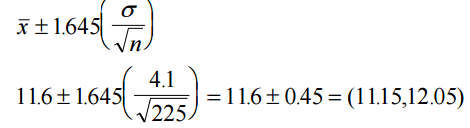#### How to Interpret the confidence intervals?

Suppose we took many samples and built a confidence interval from each sample using the above equation. Then about 90% of those intervals would contain the actual mean, µ.  This is the correct interpretation of confidence interval.

So, we can say for the above example, 90% refers to the percentage of all possible intervals that contain μ i.e. to the estimation process rather than a particular interval.

It is incorrect to say that there is a probability of 0.90 that μ is between 11.15 and 12.05. In fact this probability is either 1 or 0 (μ either is or is not in the interval).

It is also incorrect to say that 90% of all tutorials had between 11.15 and 12.05 missing students.

#### Example 2:

A sample of 50 college students were asked how many exclusive relationships they’ve been in so far. The students in sample had an average of 3.2 exclusive relationship, with a standard deviation of 1.74. In addition, the sample distribution was slightly skewed to the right. Estimate the true average number of exclusive relationship based on this sample using 95% confidence interval.

So,

n=50

¯x = 3.2

S= 1.74

We assume that the number of exclusive relationships one student in the sample has been in is independent of another. So, it is random and it is < 10% of all college student. On the other hand, n> 30 and not so skewed. So, it is normal distribution and  it meets all required the conditions for calculating confidence interval.

First, we need to calculate the standard error(SE). Since we need this value to calculate margin of error.

SE== 1.74/SQRT(50) = 0.246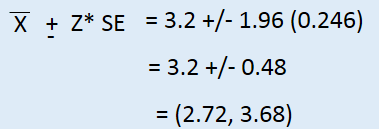It means that we are 95% confident that college student on average have been in 2.72 to 3.68 exclusive relationship.